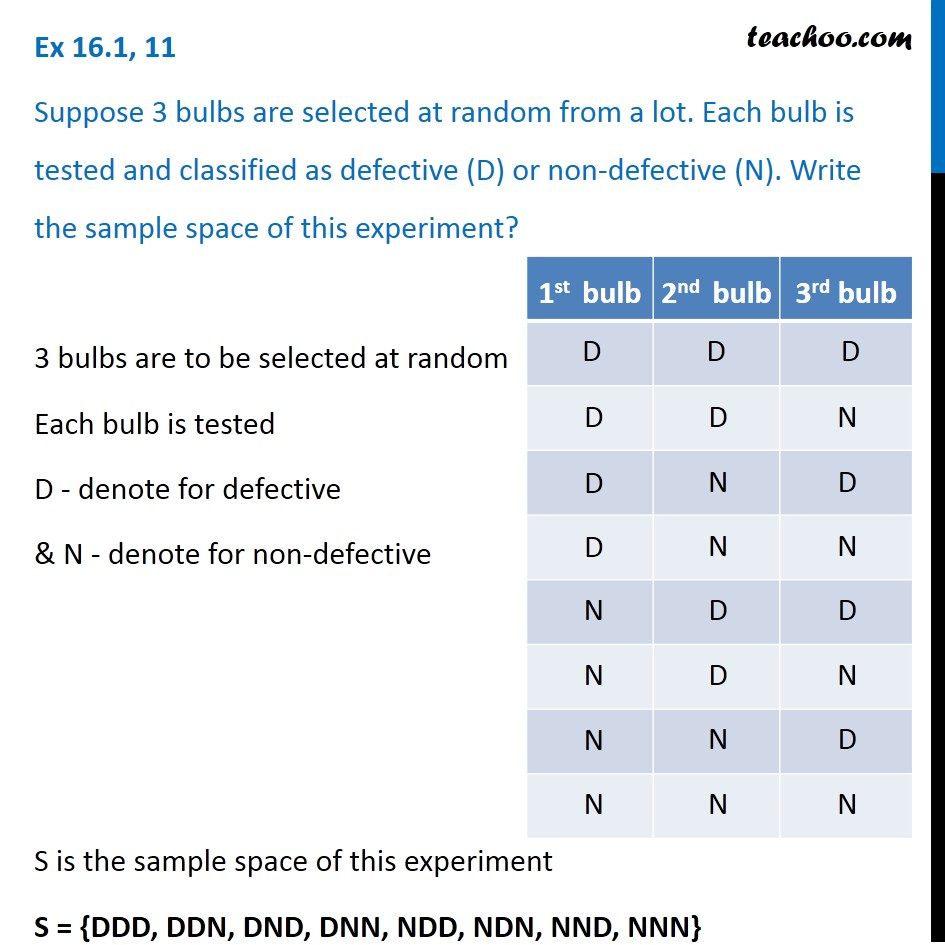Ex 16.1

Chapter 16 Class 11 Probability (Term 2)
Serial order wise### Transcript

Ex 16.1, 11 Suppose 3 bulbs are selected at random from a lot. Each bulb is tested and classified as defective (D) or non-defective (N). Write the sample space of this experiment? 3 bulbs are to be selected at random Each bulb is tested D - denote for defective & N - denote for non-defective S is the sample space of this experiment S = {DDD, DDN, DND, DNN, NDD, NDN, NND, NNN}